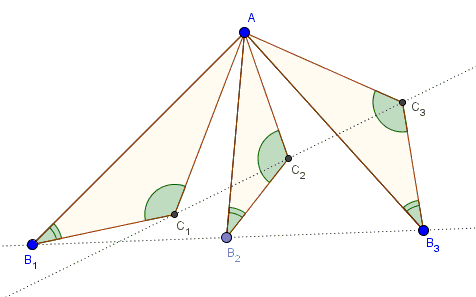# Spiral Similarity and Collinearity

The following problem has been posted by Dao Thanh Oai at the CutTheKnotMath facebook page

Assume triangles $AB_1C_1,$ $AB_2C_2,$ and $AB_3C_3$ are similar. Then, if $B_1B_2B_3$ are collinear so are $C_1C_2C_3.$Below is a dynamic illustration of the concept:

The statement could be derived with some effort from the Fundamental Theorem of Directly Similar Figures. But using the same technique (complex numbers) the explanation is straightforward.

Let's place $A$ at the origin and use $p$ as the complex number corresponding to $B_1.$ Choose any two complex numbers, say, $u$ and $v$. Recollect that multiplication by a complex number amounts to a rotation (corresponding to its argument) combined with a homothety (corresponding to its modulus), i.e. a spiral similarity. Assume $p,$ $up,$ and $vp$ are collinear, which is expressed as, say:

$\displaystyle\frac{p-up}{p-vp} \space\mbox{is a real number}.$

However, $\displaystyle\frac{p-up}{p-vp}=\frac{1-u}{1-v}$ independent of $p$ such that if $p,$ $up,$ and $vp$ are collinear for one $p,$ they are collinear for any other.

To solve the problem stated at the top of the page, observe that for the three similar triangles, if $B_2$ and $B_3$ are obtained by two spiral similarities from $B_1$ then it is also true that $C_2$ and $C_3$ are obtained by the same two spiral similarities from $C_1.$ In fact, this is true for all corresponding points in the triangles: midpoiints of sides, centroids, orthocenters, circumcenters, etc.### Geometric Transformations